start page | rating of books | rating of authors | reviews | copyrights

# 3. Data Types

Contents:
Primitive Types
Reference Types

A data type defines the set of values that an expression can produce or a variable can contain. The data type of a variable or expression also defines the operations that can be performed on the variable or expression. The type of a variable is established by the variable's declaration, while the type of an expression is determined by the definitions of its operators and the types of their operands.

Conceptually, there are two types of data in Java programs: primitive types and reference types. The primitive types are self-contained values that can be contained in a variable. The primitive types are comprised of integer types, floating-point types, and the boolean type. Of these, the integer types and floating-point types are considered arithmetic types, since arithmetic can be performed on them. Reference types contain values that point to or identify arrays or objects. The syntax for specifying a type is: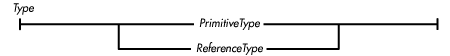## 3.1 Primitive Types

A primitive data type represents a single value, such as a number, a character, or a Boolean value. Java has primitive types for arithmetic and Boolean data: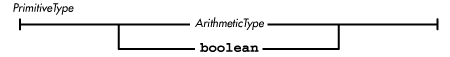References Arithmetic Types; Boolean Type

### Arithmetic Types

Unlike in C/C++, all of the arithmetic data types in Java are specified to have representations that are independent of the particular computer running a Java program. This guarantees that numeric computations made by Java programs produce the same results on all platforms.

There are two kinds of arithmetic types: integer and floating-point.

The integer types are: byte, short, int, long, and char. Like C/C++, character data is considered an integer type because of its representation and because arithmetic operations can be performed on char data. Unlike C/C++, however, short int and long int are not valid data types in Java. In addition, signed and unsigned do not have any special meaning in Java.

The floating-point data types are float and double.

The formal definition of an arithmetic type is: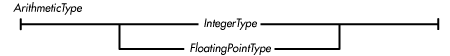References Integer types; Floating-point types

#### Integer types

Java provides integer data types in a variety of sizes. Unlike C/C++, however, the sizes of these types are part of the language specification; they are not platform-dependent. Formally: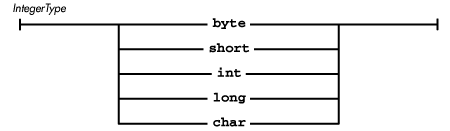The values represented by these types are specified in Table 3-1. The representation shown is used on all platforms and is independent of the native platform architecture.

Table 3.1: Integer Types and Their Representations

Type

Representation

Range

byte

8-bit, signed, two's complement

-128 to 127

short

16-bit, signed, two's complement

-32768 to 32767

int

32-bit, signed, two's complement

-2147483648 to 2147483647

long

64-bit, signed, two's complement

-9223372036854775808 to 9223372036854775807

char

16-bit, unsigned, Unicode

'\u0000' to '\uffff'

All of the signed integer types in Java use a two's complement representation. Two's complement is a binary encoding for integers, which has the following properties:

• The leftmost bit is the sign bit. If the sign bit is 1, the number is negative.

• Positive numbers have the usual binary representation.

• Negating a number involves complementing all of the bits in the number and then adding 1 to the result.

• The most negative value does not have a positive equivalent.

The java.lang package includes the Byte, Short, Integer, Long, and Character classes. These classes provide object wrappers for byte, short, int, long, and char values, respectively. Each of these classes defines static MIN_VALUE and MAX_VALUE variables for its minimum and maximum values.

Java performs all integer arithmetic using int or long operations. A value that is of type byte, short, or char is widened to an int or a long before the arithmetic operation is performed.

A value of any integer type can be cast (i.e., converted) to a value of any other integer type. Integer types, however, cannot be cast to a boolean value, nor can the boolean type be cast to an integer-type value. A value of a signed integer type can be assigned to a value of the same or wider type without a cast. In this case, the value is automatically widened to the appropriate type. Table 3-2 shows whether an assignment from a particular integer type to another integer type can be done directly or if it requires a type cast.

Table 3.2: Assignment Compatibility Between Integer Types

To/From

byte

char

short

int

long

byte

Assignable

Cast needed

Cast needed

Cast needed

Cast needed

char

Cast needed

Assignable

Cast needed

Cast needed

Cast needed

short

Assignable

Cast needed

Assignable

Cast needed

Cast needed

int

Assignable

Assignable

Assignable

Assignable

Cast needed

long

Assignable

Assignable

Assignable

Assignable

Assignable

The principle underlying the above table is that assignments that do not lose information do not require a type cast. Assigning a short value to an int without a cast is allowed because all of the values that can be represented by a short can also be represented by int. However, assigning an int value to a short is not allowed without a cast because it involves going from a 32-bit signed quantity to a 16-bit signed quantity. Similarly, a byte value cannot be assigned to char without a cast. byte is an 8-bit signed quantity, so it can represent negative numbers. However, char is a 16-bit unsigned quantity, so it cannot represent negative numbers.

Java provides the following kinds of operators for integer values:

• Comparison operators

• Arithmetic operators

• Increment and decrement operators

• Bitwise logical operators

If all of the operands of an operator are of an integer type, the operation is performed as an integer operation. Normally, integer operations are performed with a precision of 32 bits. If at least one of the operands of an integer operation is a long, however, the operation is performed with a precision of 64 bits.

When an integer operation overflows or underflows, there is no indication given that the overflow or underflow occurred.

If the right-hand operand (the divisor) of a division or remainder operation is 0, Java throws an ArithmeticException. Division by zero is the only circumstance that can cause an integer operation to throw an exception.

#### Floating-point types

Like C/C++, Java provides two sizes of floating-point numbers: single precision and double precision.

Formally: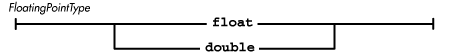Java uses the single precision 32-bit IEEE 754 format to represent float data and the double precision 64-bit IEEE 754 format to represent double data. These representations are used on all platforms, whether or not there is native support for the formats. The values represented by these types are shown in Table 3-3.

 The IEEE 754 floating-point data representation and operations on it are defined in IEEE Standard for Binary Floating-Point Arithmetic, ANSI/IEEE Std. 754-1985 (IEEE, New York). The standard can be ordered by calling (908) 981-0060 or writing to IEEE, 445 Hoes Lane, PO Box 1331, Piscataway, NJ 08855-1331, USA.

Table 3.3: Floating-Point Types and Their Representations

Type

Representation

Range

float

32-bit, IEEE 754

1.40239846e-45 to 3.40282347e+38

double

64-bit, IEEE 754

4.94065645841246544e-324 to 1.79769313486231570e+308

Normally, non-zero float values are represented as:

```sign*mantissa*2^exponent
```

where sign is +1 or -1, mantissa is a positive integer less than 2^24, and exponent is an integer in the inclusive range -149 to 104.

Non-zero double values are represented as:

```sign*mantissa*2^exponent
```

where sign is +1 or -1, mantissa is a positive integer less than 2^53, and exponent is an integer in the inclusive range -1045 to 1000.

In addition, the IEEE 754 standard defines three special values:

Positive infinity

This value is produced when a float or double operation overflows, or a positive value is divided by zero. Positive infinity is by definition greater than any other float or double value.

Negative infinity

This value is produced when a float or double operation overflows, or a negative value is divided by zero. Negative infinity is by definition less than any other float or double value.

Not-a-number (NaN)

This value is produced by the float and double operations such as the division of zero by zero. When NaN is one of the operands for an operation, most arithmetic operations return NaN as the result. Since NaN is unordered, most comparison operators (e.g., <, <=, ==, >=, >) return false when one of their arguments is NaN. The exception is !=, which returns true when one of its arguments is NaN.

The java.lang package includes Float and Double classes that provide object wrappers for float and double values. Each class defines the three special values as symbolic constants: POSITIVE_INFINITY, NEGATIVE_INFINITY, and NaN. Each class also defines MIN_VALUE and MAX_VALUE constants for its minimum and maximum values.

Floating-point operations never throw exceptions. Operations that overflow produce positive or negative infinity. Operations that underflow produce positive or negative zero. Operations that have no defined result produce not-a-number.

Both float and double data types have distinct representations for positive and negative zero. These values compare as equal (0.0 == -0.0). Positive and negative zero do produce different results for some arithmetic operations, however: 1.0/0.0 produces positive infinity, while 1.0/-0.0 produces negative infinity.

A float value can be assigned to a double variable without using a type cast, but assigning a double value to a float variable does require a cast. Conversion from a float or double value to any other data type also requires a cast. Either of the floating-point data types can be cast to any other arithmetic type, but they cannot be cast to boolean. When a floating-point number is cast to an integer type, it is truncated (i.e., rounded toward zero).

Java provides the following kinds of operators for floating-point values:

• Comparison operators

• Arithmetic operators

• Increment and decrement operators

If any of the arguments of an operation are of a floating-point type, the operation is performed as a floating-point operation. In other words, any of the integer operands are converted to floating point before the operation takes place. Floating-point operations are normally performed with a precision of 32 bits. However, if at least one of the operands of the operation is a double, the operation is performed with a precision of 64 bits.

### Boolean Type

The boolean data type represents two values: true and false. These values are keywords in Java. The java.lang package includes a Boolean class that provides an object wrapper for boolean values. This Boolean class defines the constant objects Boolean.TRUE and Boolean.FALSE.

Java provides the following kinds of operators for boolean values:

• Equality and inequality operators

• Boolean logical operators

The following Java constructs require a boolean value to specify a condition:

• if

• while

• for

• do

• The conditional operator ? :

Unlike C/C++, any attempt to substitute a different type for boolean in these constructs is treated as an error by Java.

No other data type can be cast to or from boolean. In particular, using the integer 1 to represent true and 0 to represent false does not work in Java. Though Java does not provide conversions between boolean and other types, it is possible to provide explicit logic to accomplish the same thing:

```int i;
i != 0             // This is true if i is not equal to zero
boolean b;
b ? 1 : 0          // If b is true produce 1; otherwise 0
```